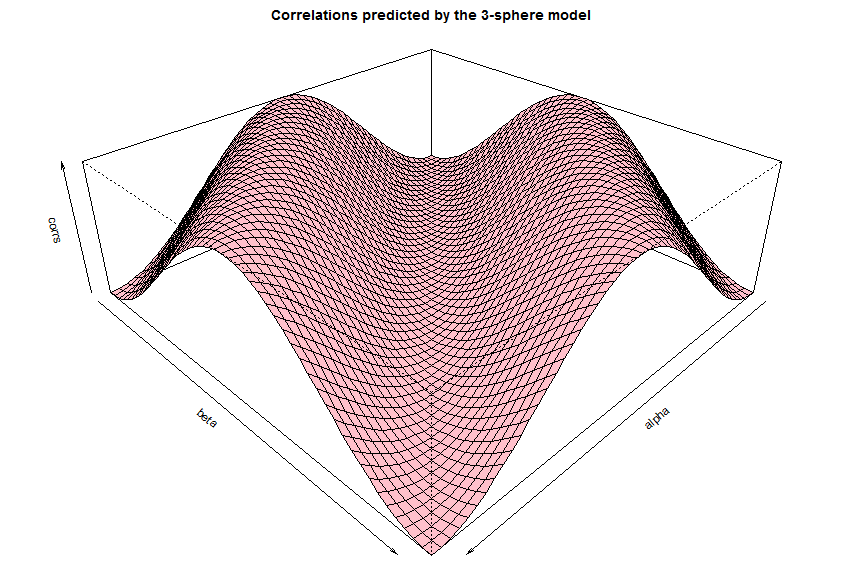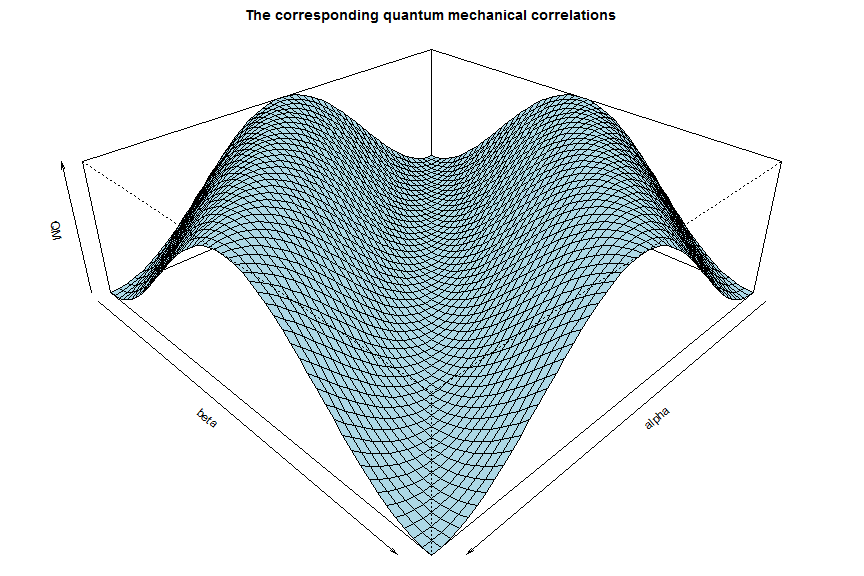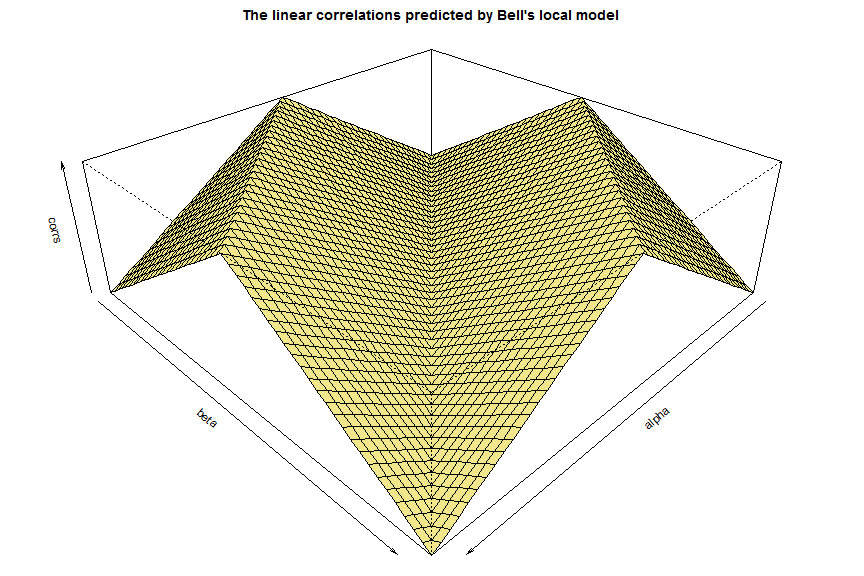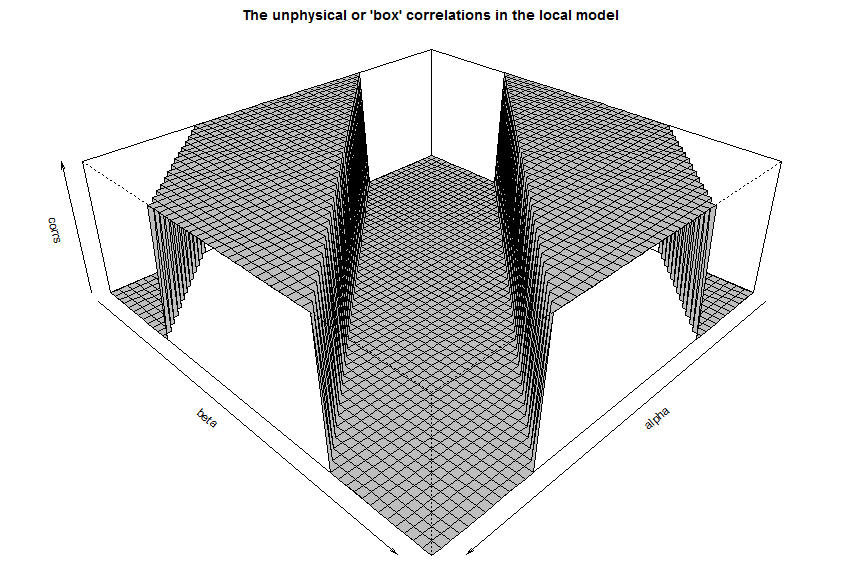``````## A simulation of my 3-sphere model for the EPR-Bohm correlation. It should
## be viewed as a supplement to the one built in http://rpubs.com/jjc/13965.
## Further details and extensive discussion of the model can be found on my
## blog: http://libertesphilosophica.info/blog/. The code for the surfaces
## plotted below has been adapted from a similar simulation by Ch'an Satori.

## The theoretical description of the model can be found in this paper:

## This is S^2 version (aka 3D version: think of S^2 as a surface in R^3)
## This version has been adapted from Richard Gill's optimized version of
## Michel Fodje's original simulation of the model, which can be found here:
## http://rpubs.com/gill1109/EPRB3opt. Later Richard Gill improved his 3D
## version by employing the exact probability distribution derived by Philip
## Pearle in his classic 1970 paper: http://rpubs.com/gill1109/Pearle. It
## should be noted, however, that, unlike Pearle's model, the 3-sphere model
## has nothing whatsoever to do with data rejection or detection loophole.

## All of the above simulations are inspired by the original simulation of
## the 3-sphere model by Chantal Roth, https://github.com/chenopodium/JCS2.

## The pink surface below represents the correlation predicted by my 3-sphere
## model, the blue surface represents the corresponding prediction of quantum
## mechanics, the yellow surface represents Bell's local model of 1964, and
## gray surface represents the maximally strong (unphysical) box correlation.

set.seed(9875)

M <- 10^5  ## Size of the pre-ensemble. Next, try 10^6 ...

angles = seq(from = 0, to = 360, by = 7.2) * pi/180

K <- length(angles)

corrs = matrix(nrow = K, ncol = K, data = 0)  ## Container for correlations

z <- runif(M, -1, 1)
t <- runif(M, 0, 2 * pi)
r <- sqrt(1 - z^2)
x <- r * cos(t)
y <- r * sin(t)

u <- rbind(x, y, z)  ## A 3xM matrix. The M columns of u represent the x, y,
## and z coordinates of M uniformly distributed points on the 2-sphere, S^2.
## For a demonstration, see, for example, http://rpubs.com/gill1109/13340.

eta <- runif(M, 0, pi)  ## My initial eta_o, or Michel Fodje's 't'

f <- -1 + (2/(sqrt(1 + ((3 * eta)/pi))))  ## Pearle's 'r' is arc cosine of 'f'

for (i in 1:K) {
alpha <- angles[i]
a <- c(cos(alpha), sin(alpha), 0)  ## Measurement direction 'a'

for (j in 1:K) {
beta <- angles[j]
b <- c(cos(beta), sin(beta), 0)  ## Measurement direction 'b'

ua <- colSums(u * a)  ## Inner products of 'u' with 'a'
ub <- colSums(u * b)  ## Inner products of 'u' with 'b'

good <- abs(ua) > f & abs(ub) > f  ## Sets the topology to that of S^3

o <- x[good]
p <- y[good]
q <- z[good]

v <- rbind(o, p, q)  ## N spin directions produced at the source

g <- f[good]  ## The initial state of spin is defined by the pair (v, g)

N <- length(v)/3  ## The number of complete states (v, g) at the source

va <- colSums(v * a)  ## Inner products of 'v' with 'a'
vb <- colSums(v * b)  ## Inner products of 'v' with 'b'

corrs[i, j] <- sum(sign(va) * sign(-vb))/N

## corrs[j] <- sum(sign(vb))/N

}
}

(N)
``````
``````##  66504
``````
``````par(mar = c(0, 0, 2, 0))
persp(x = angles, y = angles, main = "Correlations predicted by the 3-sphere model",
z = corrs, zlim = c(-1, 1), col = "pink", theta = 135, phi = 30, scale = FALSE,
xlab = "alpha", ylab = "beta")
````````````par(mar = c(0, 0, 2, 0))

QM = matrix(nrow = K, ncol = K, data = sapply(angles, function(t) -cos(t - angles)),
byrow = TRUE)
persp(x = angles, y = angles, main = "The corresponding quantum mechanical correlations",
z = QM, zlim = c(-1, 1), col = "lightblue", theta = 135, phi = 30, scale = FALSE,
xlab = "alpha", ylab = "beta")
````````````par(mar = c(0, 0, 2, 0))

f = 0  # Switching the topology from S^3 to R^3.

for (i in 1:K) {
alpha = angles[i]
a = c(cos(alpha), sin(alpha), 0)  ## Measurement direction 'a'
for (j in 1:K) {
beta = angles[j]
b = c(cos(beta), sin(beta), 0)  ## Measurement direction 'b'
ua <- colSums(u * a)  ## Inner products of 'u' with 'a'
ub <- colSums(u * b)  ## Inner products of 'u' with 'b'
good <- abs(ua) > f & abs(ub) > f  ## Sets the topology to that of S^3
o <- x[good]
p <- y[good]
q <- z[good]
v <- rbind(o, p, q)  ## N spin directions produced at the source
N <- length(v)/3  ## The number of initial states v at the source
va <- colSums(v * a)  ## Inner products of 'v' with 'a'
vb <- colSums(v * b)  ## Inner products of 'v' with 'b'

corrs[i, j] <- sum(sign(va) * sign(-vb))/N

## corrs[j] <- sum(sign(vb))/N

}
}

persp(x = angles, y = angles, main = "The linear correlations predicted by Bell's local model",
z = corrs, zlim = c(-1, 1), col = "khaki", theta = 135, phi = 30, scale = FALSE,
xlab = "alpha", ylab = "beta")
````````````par(mar = c(0, 0, 2, 0))

f = 0.7  # Switching the topology from S^3 to stronger-than-S^3

for (i in 1:K) {
alpha = angles[i]
a = c(cos(alpha), sin(alpha), 0)  ## Measurement direction 'a'
for (j in 1:K) {
beta = angles[j]
b = c(cos(beta), sin(beta), 0)  ## Measurement direction 'b'
ua <- colSums(u * a)  ## Inner products of 'u' with 'a'
ub <- colSums(u * b)  ## Inner products of 'u' with 'b'
good <- abs(ua) > f & abs(ub) > f  ## Sets the topology to that of S^3
o <- x[good]
p <- y[good]
q <- z[good]
v <- rbind(o, p, q)  ## N spin directions produced at the source
N <- length(v)/3  ## The number of initial states v at the source
va <- colSums(v * a)  ## Inner products of 'v' with 'a'
vb <- colSums(v * b)  ## Inner products of 'v' with 'b'

corrs[i, j] <- sum(sign(va) * sign(-vb))/N

## corrs[j] <- sum(sign(vb))/N

}
}

persp(x = angles, y = angles, main = "The unphysical or 'box' correlations in the local model",
z = corrs, zlim = c(-1, 1), col = "gray", theta = 135, phi = 30, scale = FALSE,
xlab = "alpha", ylab = "beta")
````````````# Further details on my blog: http://libertesphilosophica.info/blog/.
``````